# Non-Contiguous Memory Allocation

Tom Kelliher, CS42

Oct. 28, 1996

Problems with contiguous allocation:

1. Problems with fixed partitions:
1. Limited degree of multiprogramming.

2. Internal fragmentation.

3. Placement policies.

2. Problems with variable partitions:
1. External fragmentation, compaction.

2. Swapping is difficult, if not impossible (address binding).

Alternative: non-contiguous allocation.

# Paging

The idea:

1. Entire process in memory.

2. Partition memory into frames of size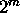.
1. Typical frame sizes: 512 to 8K bytes.

2. Frame size constrained by MMU design.

3. Logical address space is broken into pages.
1. Page size = frame size.

2. Pages can be arbitrarily mapped onto frames.

3. However, process ``sees'' a contiguous, flat address space.

1. Assume logical address is n bits.

2. Page number field is n - m most significant bits.

3. Page offset field is m least significant bits.

4. Using table look-up, convert logical page number to physical frame number.

5. Frame offset = page offset.

Why is frame, page size a power of two?

Paging hardware: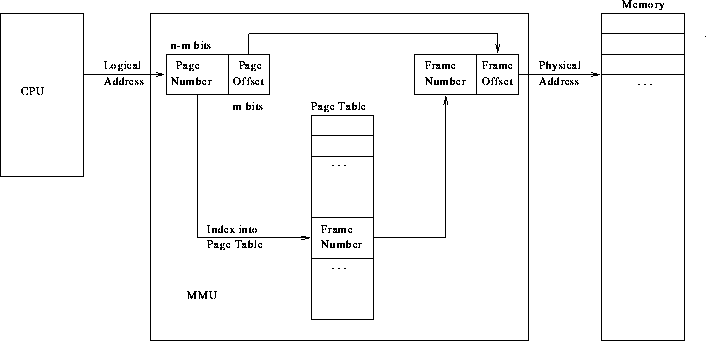Design issues:

1. Page size:
1. Internal fragmentation.

2. Maximizing I/O transfer rate.

2. I/O --- process passes logical address to kernel.

3. Implementation of the page table.
1. Small register file.

2. Array in memory.

4. Issues for memory implementation:
1. Page table must be in contiguous memory.

2. Page table base register.

3. ``Logical memory access'' requires two physical accesses.
1. Translation look-aside buffer.

2. TLB entries contain: Page number, frame number pairs.

3. Issue: Context switches.

4. Only a few entries needed.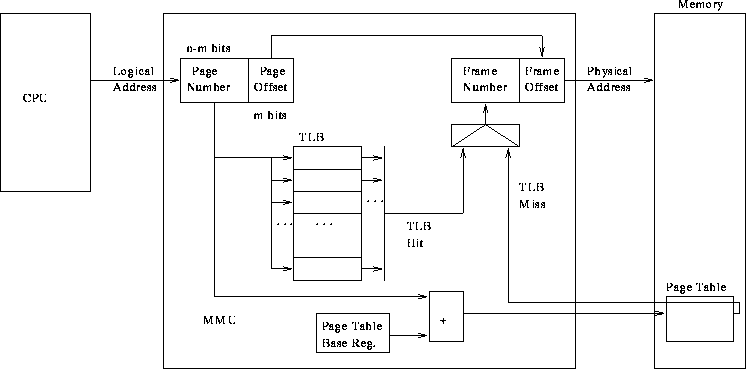## Size, Structure of Page Table

1. Problem: huge page tables. How did this happen?

2. Solutions:
1. Valid/invalid bit.

2. Page table limit register.

3. Multi-level paging.

### Multi-Level Paging

Why not just use the limit register?

A two-level paging scheme for a 32-bit logical address: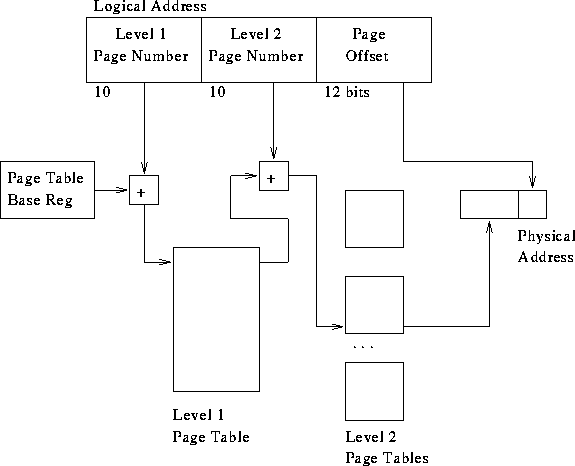Is the page table really any smaller?

What does this solve?

1. Non-contiguous (paged) page table.

2. ``Holes'' in page table (valid bit) shrink it.

Sun SPARC: 3 level paging.

## Protection

1. Is it possible for a process to access an arbitrary memory location?

2. Using valid bit to introduce ``holes'' into logical address space.

## Page Sharing

1. What can be shared?

3. Page alignment --- segment the logical address space.

4. Page de-allocation.

# Segmentation

1. ``Object oriented'' approach:
1. User views program as a set of objects:
1. Stack.

2. Routines.

3. Arrays.

4. ...

2. Each object stored in a segment.

2. Generalization of paging.

3. Logical address is a segment ``name'' and an offset.

4. Variable-sized ``page'' having a base and length.

5. Frames start onmemory boundaries. Segments start anywhere. Therefore, must add a segment base and offset.

6. Straight paging: external fragmentation.

7. Solution: Paged segmentation (x86 architecture).

Segmentation hardware: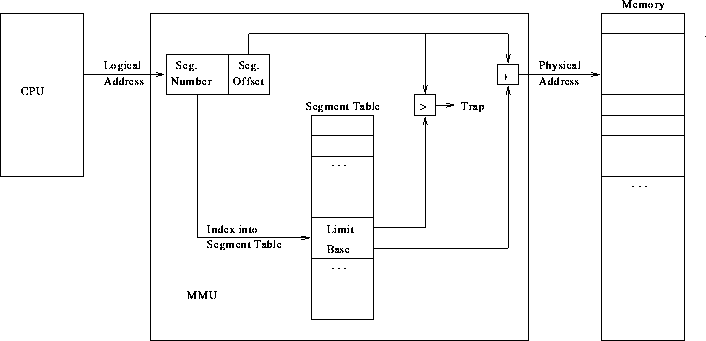Thomas P. Kelliher
Fri Oct 25 16:55:29 EDT 1996
Tom Kelliher